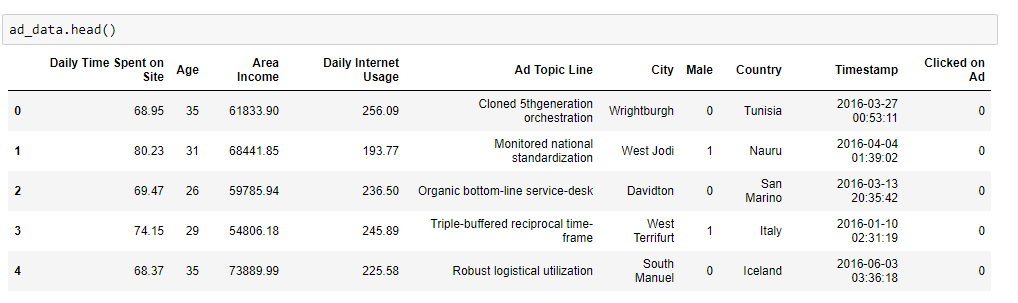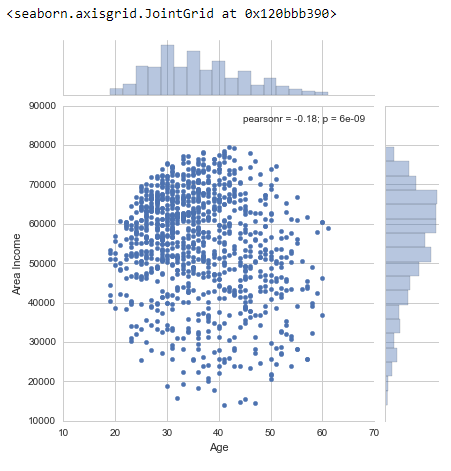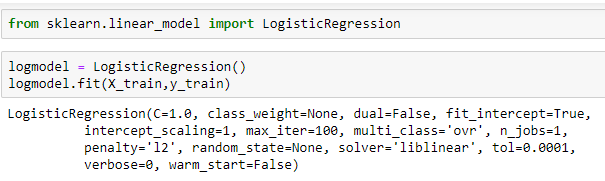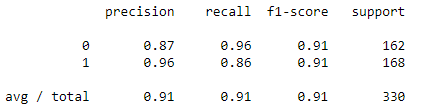# Explain with a case study of logistic regression in Python.

In order to implement a logistic regression in Python we need to import following basic libraries

import pandas as pd

import numpy as np

import matplotlib.pyplot as plt

import seaborn as sns

%matplotlib inline

Now we will import the data and explore the dataWe will visualize the data in order to explore the weightage of the dataNow we will split the data to create a model

from sklearn.model_selection import train_test_split

X=ad_data[['Daily Time Spent on Site','Age', 'Area Income','Daily Internet Usage', 'Male']]

X_train,X_test,y_train,y_test=train_test_split(X,y,test_size=0.33, random_state=42)

Now we will create and fit the modelNow we will predict the test data and evaluate the model

from sklearn.linear_model import LogisticRegression

log model = LogisticRegression()

log model.fit(X_train,y_train)

predictions = log model.predict(X_test)

from sklearn.metrics import classification_report

print(classification_report(y_test,predictions))This is how we implement logistic Regression in Python